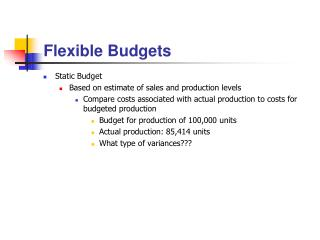DownloadDownload PresentationFlexible Budgets

# Flexible Budgets

Download Presentation## Flexible Budgets

- - - - - - - - - - - - - - - - - - - - - - - - - - - E N D - - - - - - - - - - - - - - - - - - - - - - - - - - -
##### Presentation Transcript

1. Flexible Budgets • Static Budget • Based on estimate of sales and production levels • Compare costs associated with actual production to costs for budgeted production • Budget for production of 100,000 units • Actual production: 85,414 units • What type of variances???

2. Flexible Budgets • Flexible Budget • Compare costs associated with actual production to expected costs for actual production • Before start of period: • Estimated production: 100,000 units • Budget for production of 80,000; 100,000 and 120,000 units • After end of period: • Actual production: 89,315 units • Budget for production of 89,315 units

3. Overhead Analysis • Variable Overhead Spending (Price) Variance • Assuming labor hours used to apply overhead… • VO Spending Variance: AH x (AVO Rate – SVO Rate) • AVO Rate = Actual VO / Actual Hours • Variable Overhead Efficiency (Volume) Variance • VO Spending Variance: (AH – SH) x SVO Rate

4. Overhead Analysis • Fixed Overhead Variance • Actual Fixed Overhead – Applied Fixed Overhead • Applied Fixed Overhead = SH x SFO Rate • SH for actual production • Fixed Overhead Spending (Price) Variance • FO Spending Variance: Actual Fixed Overhead – Budgeted Overhead • Budgeted Overhead = SFO Rate x Expected Hours • Expected Hours for budgeted production • Fixed Overhead Volume Variance • FO Volume Variance: Budgeted Overhead – Applied Overhead

5. Overhead Analysis • Fixed Overhead Variance • What if actual production < budgeted production • Will be under applied